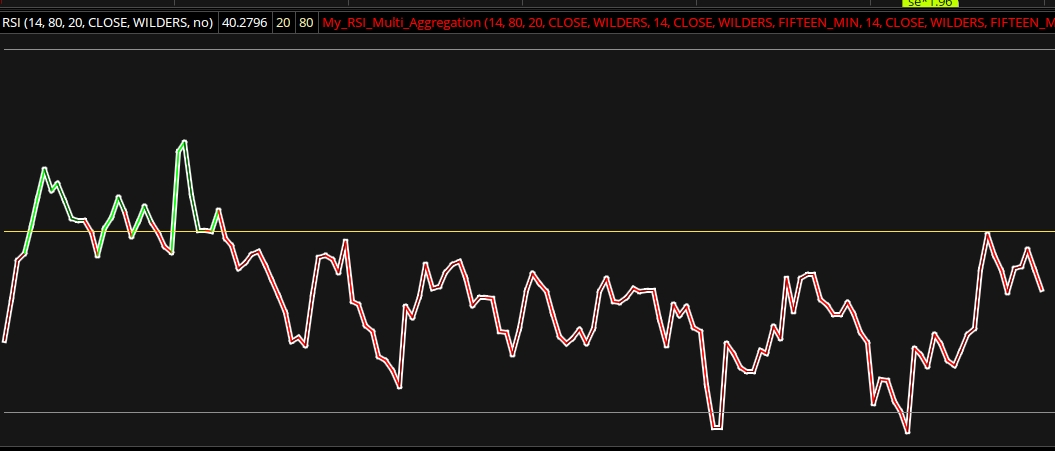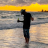# Does anyone have Multi Timeframe RSI?

J

#### john3

##### Active member
2019 Donor
Hello,

If you guys have MTF RSI please post it. If not, would somebody please code it?

Thank you.VIP
J

#### john3

##### Active member
2019 Donor
@horserider Thank you, but it doesn't seem to be correct. I'm on 8 minutes and selected to plot RSI on 15 minutes. I have a separate 15-min chart with RSI on it, the MTF RSI doesn't match the one on 15 minutes.#### horserider

##### Well-known member
VIPMTF red/green simple RSI overlay white

Last edited:
J

#### john3

##### Active member
2019 Donor
@horserider I've checked it again. It does not seem to match TOS' RSI on the same time frame.

Edit: I've found an issue and fixed it. Mobius' code uses a modified RSI not the original one, that was the issue.

Last edited by a moderator:
•horserider
A

##### New member
@horserider @BenTen

I would like to see labels for RSI (length 14) for 5, 15, 30, 60, day, wk and Month. Lable color Green > 50 line and Red <50 line. I would like to add this to my price chart. Please help. Thank you.

A

##### New member

Thank you so much Ben. Here is the script I started. But, the 1min and 5 min RSI ended up with the same value. They should be different. What am I doing wrong? Thank you.

Code:
``````#Show/hide aggregation periods
input show1m  = yes;#Hint show1m:1 minute
input show2m  = yes;#Hint show2m:2 minutes
input show3m  = yes;#Hint show3m:3 minutes
input show4m  = yes;#Hint show4m:4 minutes
input show5m  = yes;#Hint show5m:5 minutes
input show10m = yes;#Hint show10m:10 minutes
input show15m = yes;#Hint show15m:15 minutes
input show20m = yes;#Hint show20m:20 minutes
input show30m = yes;#Hint show30m:30 minutes
input show60m = yes;#Hint show60m:1 hour
input show2hr = yes;#Hint show2hr:2 hours
input show4hr = yes;#Hint show4hr:4 hours
input show1d  = yes;#Hint show1d:1 day

### 1m timeframe ###
def tf1m = AggregationPeriod.MIN;

def rsi = reference RSI("over bought" = 95, "over sold" = 5, price = close(period = AggregationPeriod.MIN));
AddLabel(yes,"RSI1m ", if rsi >= 50 or rsi <= 50 then Color.RED else Color.GREEN);

### 5m timeframe ###
def tf5m = AggregationPeriod.five_MIN;

def rsi5 = reference RSI("over bought" = 95, "over sold" = 5, price = close(period = AggregationPeriod.FIVE_MIN));
AddLabel(yes, "RSI5m", if rsi >= 50 or rsi <= 50 then Color.RED else Color.GREEN);``````#### BenTen

Staff
VIP
@ashfordtrader You probably didn't have the correct matching for each variable of the higher timeframe. Better if you post your entire script so we can take a look.

A

##### New member
Thank you so much Ben. Here is the script I started. But, the 1min and 5 min RSI ended up with the same value. They should be different. What am I doing wrong? Thank you.

Code:
``````#Show/hide aggregation periods
input show1m  = yes;#Hint show1m:1 minute
input show2m  = yes;#Hint show2m:2 minutes
input show3m  = yes;#Hint show3m:3 minutes
input show4m  = yes;#Hint show4m:4 minutes
input show5m  = yes;#Hint show5m:5 minutes
input show10m = yes;#Hint show10m:10 minutes
input show15m = yes;#Hint show15m:15 minutes
input show20m = yes;#Hint show20m:20 minutes
input show30m = yes;#Hint show30m:30 minutes
input show60m = yes;#Hint show60m:1 hour
input show2hr = yes;#Hint show2hr:2 hours
input show4hr = yes;#Hint show4hr:4 hours
input show1d  = yes;#Hint show1d:1 day

### 1m timeframe ###
def tf1m = AggregationPeriod.MIN;

def rsi = reference RSI("over bought" = 95, "over sold" = 5, price = close(period = AggregationPeriod.MIN));
AddLabel(yes,"RSI1m ", if rsi >= 50 or rsi <= 50 then Color.RED else Color.GREEN);

### 5m timeframe ###
def tf5m = AggregationPeriod.five_MIN;

def rsi5 = reference RSI("over bought" = 95, "over sold" = 5, price = close(period = AggregationPeriod.FIVE_MIN));
AddLabel(yes, "RSI5m", if rsi >= 50 or rsi <= 50 then Color.RED else Color.GREEN);``````

Thank you Ben. This is the whole scrip:

#Show/hide aggregation periods
input show1m = yes;#Hint show1m:1 minute
input show5m = yes;#Hint show5m:5 minutes

### 1m timeframe ###
def tf1m = AggregationPeriod.MIN;

def rsi = reference RSI("over bought" = 95, "over sold" = 5, price = close(period = AggregationPeriod.MIN));
AddLabel(yes,"RSI1m ", if rsi >= 50 or rsi <= 50 then Color.RED else Color.GREEN);

### 5m timeframe ###
def tf5m = AggregationPeriod.five_MIN;

def rsi5 = reference RSI("over bought" = 95, "over sold" = 5, price = close(period = AggregationPeriod.FIVE_MIN));
AddLabel(yes, "RSI5m", if rsi >= 50 or rsi <= 50 then Color.RED else Color.GREEN);#### BenTen

Staff
VIP
@ashfordtrader Here you go, take a look at this revised script:

Code:
``````#Show/hide aggregation periods

input show1m  = yes;#Hint show1m:1 minute

input show5m  = yes;#Hint show5m:5 minutes

### 1m timeframe ###

def tf1m = AggregationPeriod.MIN;

def rsi = reference RSI("over bought" = 95, "over sold" = 5, price = close(period = AggregationPeriod.MIN));

AddLabel(yes, Concat("RSI1 = ", rsi), color.orange);

### 5m timeframe ###

def tf5m = AggregationPeriod.five_MIN;

def rsi5 = reference RSI("over bought" = 95, "over sold" = 5, price = close(period = AggregationPeriod.FIVE_MIN));

AddLabel(yes, Concat("RSI5 = ", rsi5), color.orange);``````

Keep in mind that since you are using an MTF version, if you go to any timeframe higher than the timeframe indicated in your script, the labels will disappear.

A

##### New member
Thank you so much Ben. When I am on 1 min chart, I see both 1min and 5 min labels, which is great. But when I go to 5min chart, both labels disappear. I was hoping to only see 5min RSI label. Is there any way to fix that?##### Well-known member
VIP
Thank you so much Ben. When I am on 1 min chart, I see both 1min and 5 min labels, which is great. But when I go to 5min chart, both labels disappear. I was hoping to only see 5min RSI label. Is there any way to fix that?
Not really... Your 1 min chart is the culprit... You can do higher on lower but not lower on higher as far as aggregation...

See if 1 & 5 and 3 & 5 work for you...

A

##### New member
Not really... Your 1 min chart is the culprit... You can do higher on lower but not lower on higher as far as aggregation...

See if 1 & 5 and 3 & 5 work for you...

Thank you @rad14733. I can only see the 2 labels when I am on 1min chart.#### BenTenDoes anyone here use Tradovate to trade Futures contracts? Questions 12My Question is off topic if there is a topic, Does anyone like P H O N K? Questions 0